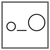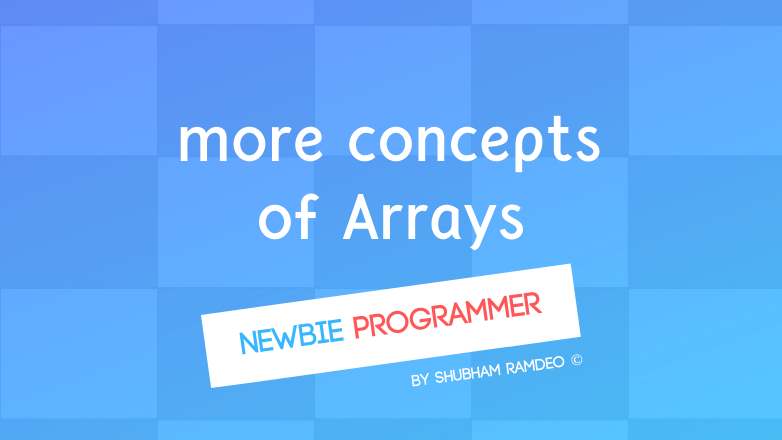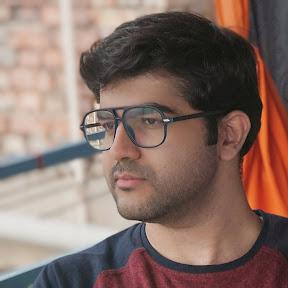Fuddlepixel coz tech is fun();

# Arrays some more concepts

Let us discuss some important concepts about arrays. The value arrays holds by default, can we change size of an array, and about character arrays.

By Shubham Ramdeo
10 March 2016 · 5 mins read
# `c`  `newbie`That’s how you play with Arrays !

Welcome to the Newbie Programmer Series. In the last part, we have introduced ourselves with a new concept called Arrays. In this part, we will take it further and will discuss some more important concepts to understand it better. So if you are new to this series, please go to index (here) and read out all the previous parts so that you can easily understand what I am talking below.

### Size of Arrays are fixed !

Whenever we make an array, we do something like this :

``````int box;
``````

This simply means that box is an integer type of arrays, which can hold 10 integers.

You can also do like this :

``````int x = 10;
int box[x];
``````

OR

``````#define X 10
...
int box[X];
``````

In all the above cases, the size of the array ‘box’ is fixed, 10 integers. We have to set the size of array. It should be fixed. The size of the array is created inside the memory of computer and remains as it is. You cannot change the size once it is created. So when you write box, the moment the program starts, a piece of memory for 10 integers will be used and will remain there until the program ends. You cannot change the size of an array while the program is running.

For example, see this piece of code below :

``````...
int x;
scanf("%d", &x);
int box[x];
...
``````

In the above code, AFTER THE PROGRAM STARTS, we are asking user to enter a number. And an array will be created of the number which the user will enter. Do you think this code will work ? NO. As we are changing the size of the array while the program is running hence this code will not work.

The size of an array is NOT VARIABLE !

So what is the solution ? Well, to create the space in the memory on the basis of user’s need, ( I mean to solve the problem with arrays ), there is a concept called Dynamic Memory Allocation. We allocate the memory dynamically while the program is running. This is a very interesting but a little advanced topic so we will discuss it later.

### Arrays needs to made empty !

Whenever we make a variable, it utilizes some unused space in the memory. That space might be unused but it doesn’t mean that the space is EMPTY. There is a lot of scrap there. So we should make all of them empty or zero.

Suppose if I make an array like this :

``````int tab;
tab = 10;
``````

Can you tell me what is the value of tab ? Well we have stated that tab is 10. But what about tab ?

Well we have not given any value, so it can be zero or any scrap value like “A^#av” OR “32811” whatever. It is not necerssory that the value will be zero. It might be anything. So we should make them zero. But how ? Like this :

``````int tab;
tab = 3;
tab = 10;
tab = 0;
tab = 0;
``````

Here we have assigned tab and tab zero so as to make them empty for our practical purpose.

But what about an array of 100 size ? Will you make each of these one hundred variables zero ? No. We can use LOOPS !

``````int data;
int x;

for(x = 0; x < 100; x++)
{
data[x] = 0;
}
``````

The above piece of code is a very important concept. The first thing is that we have made an array ‘data’ of size 100 integers. And also an integer x. Then we have used for loop to make each of this array zero.

The loop starts from x = 0. And we have discussed in detail in the previous parts that in C language, arrays starts from zero. As the size of the array is 100, it would be between data to data.

In the for loop, we have limited the loop to “ x < 100” which means highest limit of loop is “x = 99” which is also the highest limit of  ’data’ array.

So the loop will go on as “0, 1, 2,….. upto 99” and will make “data  = 0, data = 0,… upto data = 0” and hence all the values inside the array will be made zero.

### Character Arrays

Until now, whenever we have make any string, we make have make it like :

``````char name;
``````

Why ? Because strings are also a kind of array. Don’t you think that a sentence is just a long chain of character ?

Suppose If I say :

``````char name;
name = "Shubham Ramdeo";
``````

Inside the ‘name’ array, this happens :

`````` _ _ _ _ _ _ _ _ _ _ _ _ _ _  _  _  _  _
|S|h|u|b|h|a|m| |R|a|m|d|e|o|\o|\o|\o|\o|.... upto 80 characters.\
- - - - - - - - - - - - - -  -  -  -  -
0 1 2 3 4 5 6 7 8 9 10......................
``````

A long table is created. Each of the box contains one letter.

Here,

``````name is 'S'
name is 'h'
name is 'u'
...
name is 'R'
...
and so on.
``````

As the ‘name’ is of size 80, it will hold 80 characters. But I have written name = “Shubham Ramdeo” which is only about 14 characters. So all the rest becomes zero OR some scrap.

The zero in terms of characters is “\o” which is “slash o” (o for orange). This means “NO CHARACTER” in C language.

Here too, We must make all the characters empty so as too remove the scrap. We can do exactly same as above :

``````char name;
int x;

for(x = 0; x < 80; x++)
{
name[x] = '\o';
}
``````

Now, all the characters are in this ‘name’ string array are set to NONE !

We have discussed a lot of concepts here. I think its enough for now. In the next part, we will do some practical examples, involving all the concepts that we have learned about arrays. So stay connected. :)

##### Update me weekly ✉

Subscribe below and we’ll send you a weekly email summary of all new Code tutorials. Never miss out on learning about the next big thing.

#### Shubham RamdeoShubham Ramdeo is the founder of Fuddlepixel. He is a geek, a writer, involved with many technical and humanity projects.

Meet me!

#### Recent#### Related

`c`

`newbie`

Top